## Home Work TWO

1. Consider two-dimensional fluid flow in a rotating frame of reference on the f plane. Linearize the equations about a state of rest.

(a) Ignore the pressure term and determine the general solution to the resulting equations. Show that the speed of fluid parcels is constant. Show that the trajectory of the fluid parcels is a circle with radius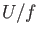, where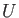is the fluid speed.

(b) What is the period of oscillation of a fluid parcel?

(c) Extra credit

If the parcel moves on a straight line in reference frame, why is the answer to (b) is not the same as a period of rotations of the frame of reference? (To answer this fully you need to understand the dynamics of inertial oscillations and inertia circles - see Durran 1993, Egger 1999, Phillips 2000.

2. Convection and its parameterization. Consider a Boussinesq system in which the vertical momentum equation is modified by the parameter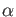to read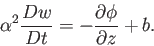and the other equations are unchanged. (If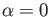the system is hydrostatic, and if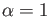the system is the original one.) Linearise these equations about a state of rest and of constant stratification and obtain the dispersion relation for the system, and plot it for various values of, including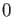and. Show that, for the case of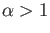the system approaches the limiting frequency of N more rapidly than with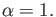Extra Credit Repeat the calculations above with added Coriolis force, i.e. by adding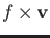term to the horizontal momentum equation.

3. Consider a rapidly rotating (i.e., in near geostrophic balance) Boussinesq fluid on the f -plane.

(a) Show that the pressure divided by the density scales as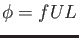.

(b) Show that the horizontal divergence of the geostrophic wind vanishes. Thus, argue that the scaling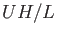is an overestimate for the magnitude of the vertical velocity. (Optional extra: obtain a scaling estimate for the magnitude of vertical velocity in rapidly rotating flow.)

(c) Using these results, or otherwise, discuss whether hydrostatic balance is more or less likely to hold in a rotating flow that in non-rotating flow.

4. On a perfectly smooth and frictionless hockey field at Dartmouth college (43 degrees North) how slowly should a puck be driven to perform an inertial circle of diameter equal to the field width (26 meters)?

Dr Yuri V Lvov 2020-02-25